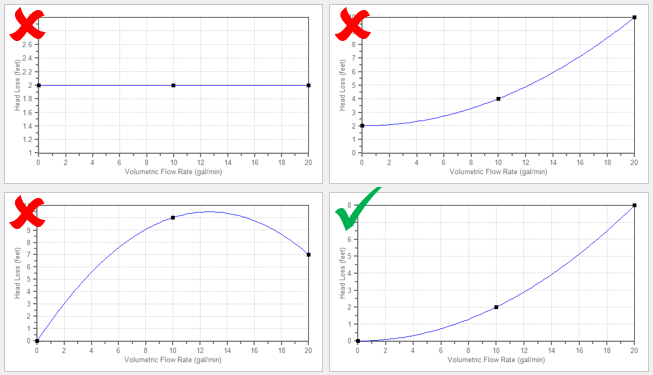## Troubleshooting Resistance Curves

Common issues with resistance curves include curves where losses do not increase with flow, and curves with a non-zero loss at zero flow. Figure 1 below shows examples of curves with potential issues compared to a correctly defined curve.Figure 1: Example resistance curves showing correct and incorrect curve types. The incorrect curves either have non-zero loss at zero flow, or have losses which do not increase with flow. The correct curve has zero loss with zero flow and has losses which increase with flow.

These common issues create two potential problems in a model.

First, if the system sees a constant pressure drop even at low flowrates, AFT Arrow's iterative solver can encounter difficulty converging on a solution since the system flow and pressure drop are no longer dependent on each other.

Second, real equipment in a system will typically cause a higher pressure drop at larger flowrates, and will not provide any loss to the system at zero flow. Users should capture a single known pressure loss data point by defining a curve which passes through two points: the known pressure drop at the tested flowrate and zero pressure drop at zero flow. The topic on Resistance Curves gives more information on defining this type of curve.